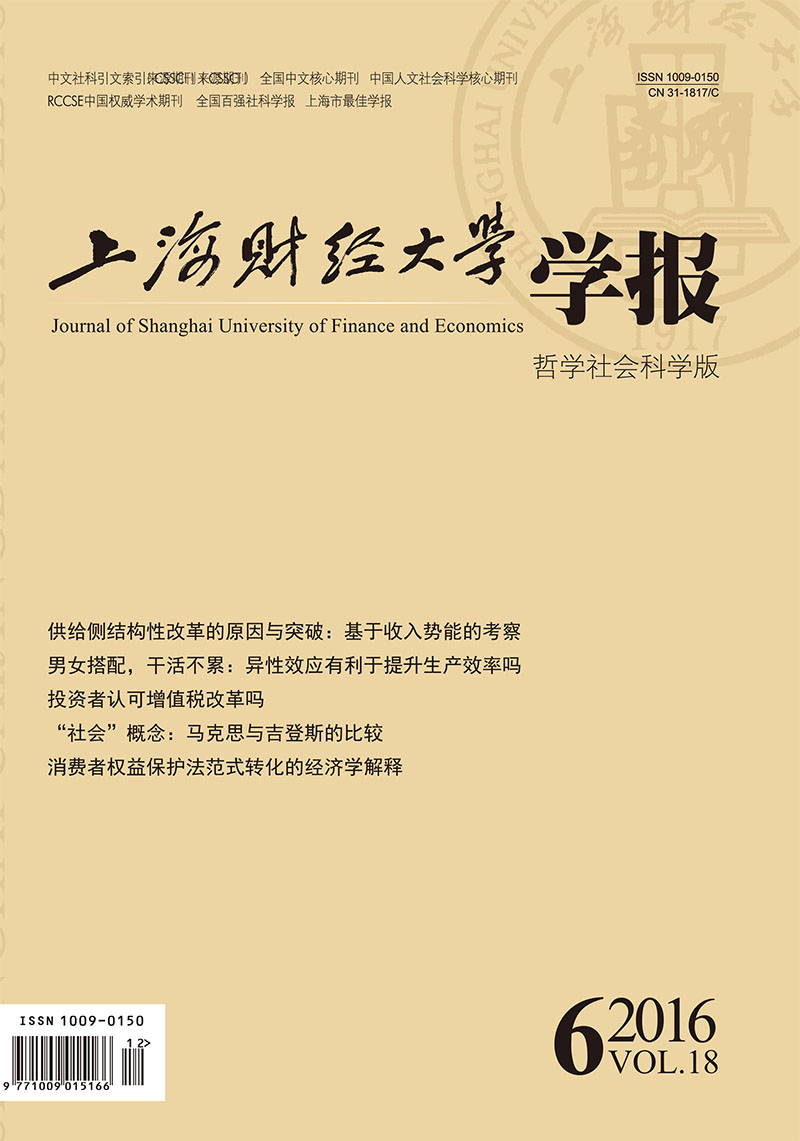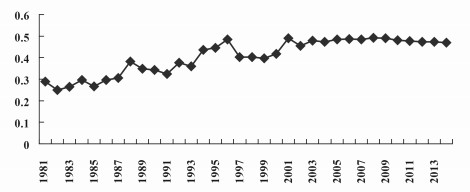﻿ 供给侧结构性改革的原因与突破:基于收入势能的考察
 上海财经大学学报2016, Vol. 18Issue (6): 4-150

#### 文章信息上海财经大学2016年18卷第6期

Zhou Weimin

Reasons for Supply-front Structural Reform and Breakthroughs:Based on the Potential Energy Model about Income

Reasons for Supply-front Structural Reform and Breakthroughs:Based on the Potential Energy Model about Income, 2016, 18(6): 4-15.

### 文章历史《上海财经大学学报》
2016第18卷第6期

1.淮阴工学院 商学院，江苏 淮安 223001;
2.香港科技大学 商学院，中国 香港

①2016年中国经济展望：多重困难挑战下如何“突围”，新华网，http://news.xinhuanet.com/fortune/2015-12/15/c_1117469687.htm，2015年12月15日。

(二) 收入势能模型

 $E_p^i = \frac{1}{2}m{v_i}^2$ (1)

 $E_c^i = \frac{1}{2}e{d_i}^2$ (2)

 $E_r^i = GMm/{d_i}^2$ (3)

 ${E_i} = \frac{1}{2}m{v_i}^2-\frac{1}{2}e{d_i}^2 + GMm/{d_i}^2$ (4)

 ${E_i} = \alpha {v_i}^2 + \beta {d_i}^2 + \gamma \frac{1}{{{d_i}^2}}$ (5)

 $\bar E = \alpha {V_E}^2 + \beta \;{\bar d^2} + \gamma \frac{1}{{{{\bar d}^2}}}$ (6)

 $E = \sum\limits_i {{E_i}^\prime } = \sum\limits_i^n {{E_i}}-n\bar E$ (7)

 $E = \sum\limits_{i = 1}^n {\left[{\alpha \left( {{V_i}^2-{V_E}^2} \right) + \beta \left( {{d_i}^2-{{\bar d}^2}} \right) + \gamma \left( {\frac{1}{{{d_i}^2}}-\frac{1}{{{{\bar d}^2}}}} \right)} \right]}$ (8)

①物理学界通常取值G=6.672 59×10-11 N•m2/kg2

(二) 中等收入陷阱缘于收入势能重回最小期望参量的过程太长图 1 中国1981-2014年历年基尼系数图 2 中国1981-2014年GDP增长率和劳动生产率增长率

(二) 推进供给侧结构性改革的内在原因解析

①参见蔡洪斌：《中国经济转型与社会流动》，载于《比较》2011年第2期第7页。

(三) 推进供给侧结构性改革的突破口选择

  蔡昉. 人口转变、人口红利与刘易斯转折点[J].经济研究,2010(4).  蔡昉. "中等收入陷阱"的理论、经验与针对性[J].经济学动态,2012(12).  蔡洪斌. 中国经济转型与社会流动[J].比较,2011(2).  钞小静, 沈坤荣. 城乡收入差距、劳动力质量与中国经济增长[J].经济研究,2014(6).  都阳, 蔡昉, 屈小博, 程杰. 延续中国奇迹:从户籍制度改革中收获红利[J].经济研究,2014(8).  樊纲, 张晓晶. "福利赶超"与"增长陷阱":拉美的教训[J].管理世界,2008(9).  贺大兴, 姚洋. 不平等、经济增长和中等收入陷阱[J].当代经济科学,2014(5).  胡鞍钢, 周绍杰, 任皓. 供给侧结构性改革--适应和引领中国经济新常态[J].清华大学学报(哲学社会科学版),2016(2).  刘伟. 突破"中等收入陷阱"的关键在于转变发展方式[J].上海行政学院学报,2011(1).  刘小梅, 刘裕. 经济发展中的城乡势能分析[J].财经科学,2004(6).  楼继伟. 中国经济的未来15年风险、动力和政策挑战[J].比较,2010(6).  马晓河. 迈过"中等收入陷阱"的需求结构演变与产业结构调整[J].宏观经济研究,2010(11).  沈坤荣. 供给侧结构性改革是经济治理思路的重大调整[J].南京社会科学,2016(2).  田雪原. 警惕人口城市化中的"拉美陷阱"[J].宏观经济研究,2006(2).  余泳泽, 武鹏. FDI、技术势能与技术外溢--来自我国高技术产业的实证研究[J].金融研究,2010(11).  张德荣. "中等收入陷阱"发生机理与中国经济增长的阶段性动力[J].经济研究,2013(9).  张文标.基于势能理论的山区公路交通流随机干扰特性分析[D].广州:华南理工大学, 2011.  郑秉文. "中等收入陷阱"与中国发展道路--基于国际经验教训的视角[J].中国人口科学,2011(1).  Benabou R. Inequality, Technology and the Social Contract[A]. Aghion P., Durlauf S. Handbook of Economic Growth[C]. Edition 1, 2005, Volume 1, Chapter 25. Princeton, NJ:Elsevier, 2005:1595-1638.  Felipe J., Abdon A. M., Kumar U. Tracking the Middle-income Trap[R]. Working Paper, Levy Economics Institute, No. 715, 2012.  Gilboy G. J., Heginbotham E. The Latin Americanization of China?[A]. Current History[C]. Philadelphia, PA, 2004:256-261.  Jankowska A., Nagengast A., Perea J. R. The Product Space and the Middle-income Trap[R]. OECD Development Centre, No. 311, 2012.  Ohno K. Avoiding the Middle-income Trap:Renovating Industrial Policy Formulation in Vietnam[J]. ASEAN Economic Bulletin,2009,26(1):25–43.  Vandenberg P., Zhuang J. How can China Avoid the Middle-income Trap?[R]. Asian Development Bank, 2011.
Reasons for Supply-front Structural Reform and Breakthroughs:Based on the Potential Energy Model about Income
Zhou Weimin1,2
1.School of Business, Huaiyin Institute of Technology, Jiangsu Huai'an 223001, China;
2.School of Business, Hong Kong University of Science and Technology, Hong Kong, China
Abstract: Under the backdrop of the "new normal" of the Chinese economy, why Chinese economy in the middle-income stage should promote the supply-front structural reform and how to promote the reform, are the very important issues worthy of further study. This paper introduces the concept of potential energy about income and constructs a potential energy model about income to make an analysis of reasons for supply-front structural reform in China and the breakthroughs. The potential energy about income means the energy influencing income growth and being in the form of potential energy, which results from income potential gap between high and low income earners owing to rapid income growth. And excessively large potential energy about income can affect labor force' production enthusiasm and ultimately harm the labor productivity. According to the principle of minimum potential energy, it would reduce the coordination of group work by widening income gap. However, there is an obvious economic gap in current China and also a trend towards growing potential energy about income. As so large-scale economy, China's economic potential energy cannot maintain for a long time in the range of the minimum potential energy. Based on the discussion of reasons for the formation of middle income trap, it clarifies the breakthroughs of the advancement of the supply-front structural reform in China. It shows that structural reform is to make full use of the market power, take the increase in the mobility of labor force as the breakthrough, make element resources like labor force flow freely, reconstitute the suitable production groups, reduce group asynchrony resulting from potential energy about income, and eventually improve labor productivity and supply-front production efficiency & capabilities.
Key words: potential energy model about income     structural reform     labor productivity     middle-income trap     labor mobility